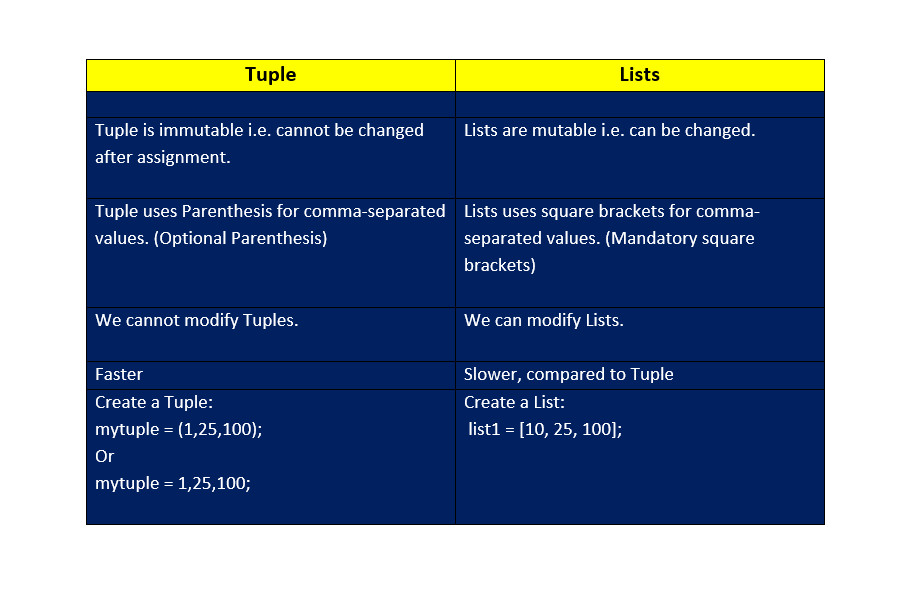## 27 May Python Tuples Tutorial with Examples

Tuple is a sequence in Python, a collection of objects. Also, Python Tuples are immutable i.e. you cannot update a tuple or any of its elements. Often, Lists and Tuples are confused in Python.  Let us see the difference between Tuple and Lists in Python.

The elements of a list can be changed after assignment, whereas this is not the case with Python Tuples. Another difference is, Tuple use parenthesis, whereas Lists in Python use Square Brackets. In other words, different comma-separated values between square brackets in a list, whereas, different comma-separated values between parenthesis is Tuple.

## Tuple vs Lists: Difference between Tuple and Lists in Python## How to create a Tuple?

To create a Tuple, set the elements inside parentheses separated by command. The parentheses are optional and feel free to remove them. Let us see an example wherein we will create a Tuple with and without using parentheses:

The output is as follows:

## Create an empty Tuple in Python

To create an empty Tuple in Python, only set two parentheses with no element in it as in the below example:

The output is as follows:

Let us now move further and perform operations on Python Tuples.

## How to access values in a Tuple?

To access a value in Tuple, set the index for which you want the value in square brackets, like:

Let us see an example to access values in Tuple:

The output is as follows:

## Check the existence of an item in a Python Tuple

The in keyword is used to check the existence of an item in a Tuple. Let us see an example:

The output is as follows:

## Indexing in Python Tuples

Indexing in Python Tuples begins from 0. The last item is tuple length – 1. Set the index in square brackets and fetch a specific element at the same index. With that, if you want to count from the right, use negative indexing. The slice operator can also be used to fetch a specific set of sub-elements. This is achieved with the colon operator and allows to fetch multiple items. Let us see them one by one.

## Fetch a specific element with indexing in Tuples

To fetch a specific element, just set the index in a square bracket i.e. refer to the index number:

Let us now see an example to fetch a specific element:

The output is as follows:

## Negative indexing in Tuples

Let to right traversal of Tuple elements are possible with negative indexing. For example, index -1 is the last item. Let us see an example of negative indexing in Tuples:

## Slicing in Tuple

The slice operator can also be used to fetch a specific set of sub-elements i.e. slicing. This is achieved with the colon operator and allows to fetch multiple items. Let us see an example:

The output is as follows:

## Range of indexes

By specifying the start and end range, we can specify the range of indexes in Python. Let us see an example:

The output is as follows:

In the above example, [1:2] means the search starts from index 1 (including) and ends at index 2 (excluding). In the same way, [1:4] means the search starts from 1 (including) and ends at index 4 (excluding).

## Range of negative indexes

To begin search from the end, set negative indexes. In a range, set negative range, such as:

Let us now see an example and set the range of negative indexes:

The output is as follows:

## Can we update Tuple values?

No, we cannot since Tuples are Immutable. However, we can convert it to list, update and then convert the list to a tuple. This will update and change the value of Tuple elements as in the example below:

The output is as follows:

## Get the total length of the Tuple

To get the total length of Tuple in Python, use the len() method:

The output is as follows:

## Concatenate two Python tuples

Let us see how to concatenate two/three tuples in Python:

The output is as follows:

## Get maximum value from Tuple

To get the maximum value from Tuple, use the max():

The output is as follows:

## Get minimum value from Tuple

To get the minimum value from Tuple, use the min():

The output is as follows:

## Convert List into Tuple

To convert List into Tuple, use the tuple(list) method and set list as the parameter. Let us see an example:

The output is as follows:

## Delete items from Tuple

Since Tuple is immutable i.e., we cannot modify/update/delete items from Tuple. However, we can delete items using the del keyword:

The output is as follows displaying error:

## Join two or more Python Tuples

The + operator is used to join two or more tuples in Python:

The output is as follows:

In this tutorial, we saw what are Tuples in Python. How Tuples are different from Lists in Python? We also saw different operations on Tuples.